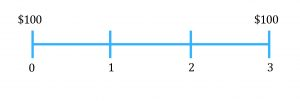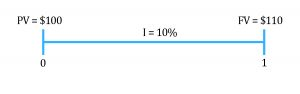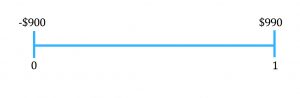Time Value of Money | IFT World
IFT Notes for Level I CFA® Program
IFT Notes for Level I CFA® Program

# Part 1

## Introductory Note

Financial Calculator: CFA Institute allows only two calculator models during the exam:

• Texas Instruments BA II Plus (including BA II Plus Professional) and
• Hewlett Packard 12C (including the HP 12C Platinum, 12C Platinum 25th anniversary edition, 12C 30th anniversary edition, and HP 12C Prestige)

Unless you are already comfortable with the HP financial calculator, we recommend using the Texas Instruments financial calculator. Explanations and keystrokes in our study materials are based on the Texas Instruments BA II Plus calculator.

Before you start using the calculator to solve problems, we recommend that you set the number of decimal places to ‘floating decimal’.

## 1. Introduction

If you have $100 today, versus an option to receive$100 after three years, what would you prefer?Obviously, you would prefer $100 today. Even though you have the same amount ($100) in both cases, you prefer $100 today. This means that there has to be some value associated with time, because you are putting more value on the$100 that you are getting today, relative to the $100 at a later point in time. This is known as ‘time value of money.’ Let us say that you are indifferent between$100 dollars today versus $110 after one year.Present value (PV): The money today or the value today is called the present value (PV = 100). This could be an investment which you make at time 0. Future value (FV): The value at a future point in time is called the future value (FV = 110). Interest rate (I): The relationship or the link between present value and future value is established through an interest rate (I = 10%). In this reading, we are essentially going to talk about these concepts: present value (PV), future value (FV), and the way we link these two concepts using interest rates (I). ## 2. Interest Rates Let’s discuss the different interpretations of interest rates using an example. Say you lend$900 today and receive $990 after one year (–ve sign indicates outflow).Interest rates can be interpreted as: 1. Required rate of return: The fact that you are willing to give$900 today on the condition that you get $990 after one year means that to engage in this transaction, you require a return of 10%. (Simple calculation will show you that the interest rate in this transaction is 10%). 2. Discount rate: You can discount the money that you will receive after one year i.e.$990 at 10% to get the present value of $900 (990/1.1 = 900). Therefore, the 10% can also be thought of as a discount rate. 3. Opportunity cost: Let’s say instead of lending the$900, you spent it on something else. You have then forgone the opportunity to earn 10% interest. Therefore, 10% can also be thought of as an opportunity cost.

Interest Rates: Investor Perspective

As an investor, we can think of the interest rate as a sum of the following components:

Let’s look at the different components.

• Real risk-free interest rate: This is the rate that you get on a security that has no risk and is extremely liquid. We make an assumption here that there is no inflation.
Example: Let’s say that you lend \$100 each to person A and person B. However, B has a high risk of default, so you are worried that he might not pay. Therefore you might demand a higher return from B as compared to A, because of the risk of default. This additional return that you demand is called the default risk premium.
• Liquidity premium: Next we have liquidity premium. This is the premium that investors demand because of the lack of liquidity of an investment.
Example: Think of two investments C and D which are similar in all regards. The only difference is that investment C is extremely liquid, whereas investment D is not that liquid. Clearly as investors, we will demand a higher return on D because it is not easy to sell. This additional return that we demand is called the liquidity premium.
• Maturity premium: Finally we have the maturity premium. This is the premium that investors demand on a security with long maturity. The maturity premium compensates investors for the increased sensitivity of the market value of debt to a change in market interest rates as maturity is extended.
Example: Let’s say we have two securities, E and F. Security E has a maturity of 1 year and security F has a maturity of 4 years. Because of the longer maturity, F has more risk, in terms of its price being more sensitive to changes in interest rate.
Instructor’s Note: You will understand this concept better when you study fixed income securities. But for now, you can take it as a given that F has higher risk because of the longer maturity.Obviously, investors will demand some compensation for the higher level of risk. This additional return that investors demand is called the maturity premium.

Nominal risk free rate:

Nominal risk-free rate = Real risk-free interest rate + Inflation premium.
So if the real risk-free rate is 3% and the inflation premium is 2%, then the nominal risk-free rate is 5%.

Instructor’s Note: On the exam if you get a term ‘risk-free rate’ with no mention of whether the rate is real or nominal, then the assumption is that we are talking about the nominal risk-free rate.

Example

 Investments Maturity (in years) Liquidity Default risk Interest Rates(%) A 1 High Low 2.0 B 1 Low Low 2.5 C 2 Low Low r D 3 High Low 3.0 E 3 Low High 4.0

1. Explain the difference between the interest rates on Investment A and Investment B.
2. Estimate the default risk premium.
3. Calculate upper and lower limits for the interest rate on Investment C, r.
1. Solution:
1. Investments A and B have the same maturity and the same default risk. However, B has a lower liquidity as compared to A. Hence, investors will demand a liquidity premium on B. The difference between their interest rates i.e. 2.5 – 2.0 = 0.5% is equal to the liquidity premium.
2. Consider investments D and E, they have the same maturity, but different liquidity and different default risk. Let’s make liquidity the same and create a new low liquidity version of D. This version will have a higher interest rate, because now investors will demand a liquidity premium. We have already determined that the liquidity premium is 0.5%. Therefore, the low liquidity version of D will have an interest rate of 3.0 + 0.5 = 3.5%.
Now compare this version of D with investment E. The only difference between the two is default risk. E has a higher default risk. Therefore, the difference between their interest rates i.e. 4.0 – 3.5 = 0.5% must be equal to the default risk premium.
3. Notice that between B and C, the only difference is that C has a longer maturity. Therefore, interest rate of C must be higher than B (2.5%).
Also notice that between C and the low liquidity version of D, the only difference is that C has a shorter maturity. Therefore, interest rate on C has to be lower than the low liquidity version of D (3.5%).
So the range for C is 2.5 < r < 3.5.

Zoom Classes for Nov-23 Exam Starting Now!
This is default text for notification bar